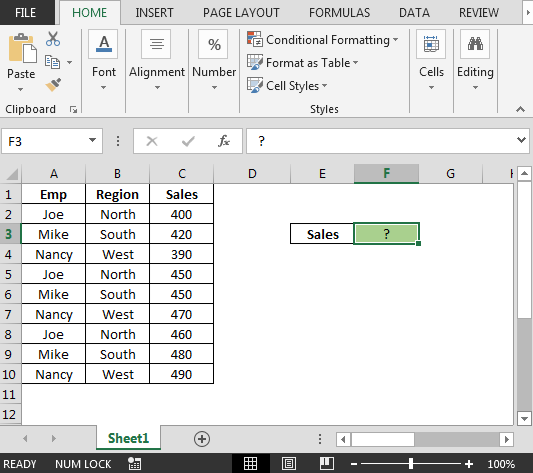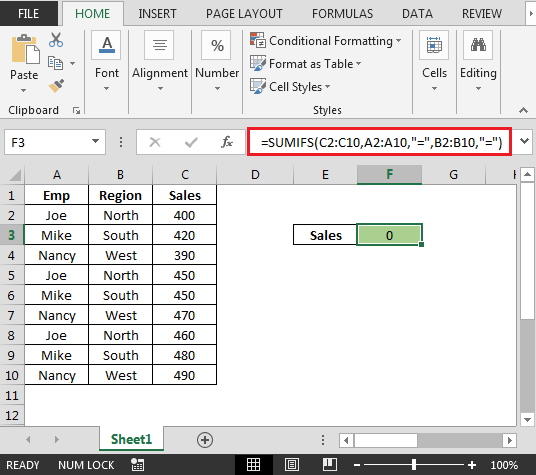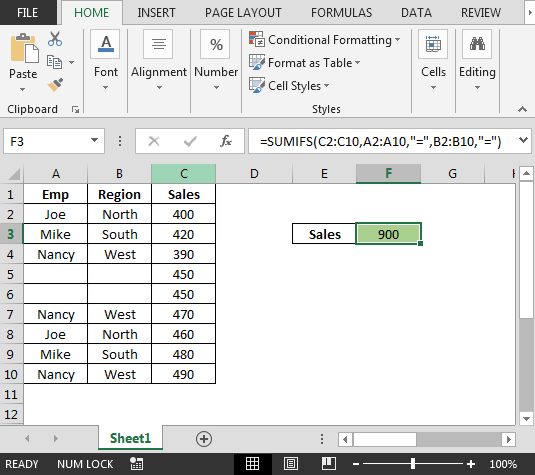# Using a Blank cell as a Criteria in Excel Sumifs

This article will use blank cell as a criteria with SUMIFS functions to calculate the Sales.

Question: I have employees’ data that contains Employee, Region & Sales in separate column. Now, I want to derive the Sales figure on the basis of the blank cells in the corresponding columns.

Following is the snapshot of data we have:The scenario is if both the columns A & B are empty then the formula should add the numbers from column C (the corresponding cells) otherwise show zero.

• In cell F3, the blank cell formula is
• `=SUMIFS(C2:C10,A2:A10,"=",B2:B10,"=")`The above formula is showing 0 since there is no blank cells in column A & B.

• If we delete cells A5:B6 then the result would be 900In this way, we can use Excel SUMIFS function to consider the blank cells as a condition.

1.Great Tip "=" & ""
use with sumifs

2.If you want to sum only if a specif range does not contain any blank cells then use this formula
=IF(COUNTBLANK(E2:E25)>0,"",SUMIF(E2:E25,"U",K2:K25) )

This will return a blank result if any of the cells in range E2:E25 is blank. Else returns the SUMIF's result.

The below article is on the same topic. Check it out. If it does not help, let me know. We will solve the problem together.

https://www.exceltip.com/logical-formulas/how-to-calculate-only-if-cell-is-not-blank-in-excel.html

3.Eg:
I am trying to Sum the amounts in Column K. Column E contains the criteria column and one of the criteria is blank cell.
I used, "sumif(E2:E25,"U",K2:K25) and "sumif(E2:E25,"",K2:K25).
I am not getting the correct answers.

I tried your comment about the custom formatting tab. It says that I should use the ones given

4.Am trying to sum a column where three criteria are met and one of the 3 has an OR operator to find 0(zero) or blank('').
Cannot get it to work. criteria 3 below is my problem. If "=0" and i put a 0 in the cell, it will work.
If null or blank, 0 does not work but "=" works. I would like to get both working. In other words empty OR zero 0.
=SUMIFS(LedgerTable[[#All],[Gross]],LedgerTable[[#All],[Month]],"="&A11,Ledger!C\$1:C\$118,'2019'!\$B\$2,LedgerTable[[#All],[Taxes]],{"=","=0"})

5.Awesome tip! Thank you!

•Yeah, and even works vice-versa "". Thanks!

6.I've tried using "=" but #value message pop out.
I want to total up in a row and if any of the cell are blank the answer shall remain Blank instead of 0 (Zero).
I.e. :
Person Round 1 Round 2 Total
Andy 70 80 150
Jessica -- -- -- (BLANK)
Ranhill -- 90 90
Ginny 90 70 160

I also tried SUM, SUMIF, SUMIFS still error.

•HI Perrier,

To solve this problem, you can simply use the sum formula along with custom formatting to display a blank space instead of zero. After applying the sum formula, select all the cells which contain the sum formula and press ctrl+ 1. Format Cells dialog box will appear, go to custom tab. You will see a label called “Type”. In the space below the TYPE label paste the following, “General;;(blank)” and then press ok. This formatting will replace all the zeros with a blank.

Happy Learning,

7.Thanks for the "=" tip! But now I have fa follow up question. Horn my sum is fordmulas, I typically have the conditions in othafer cells which I then refer to in my formula. I tried putting "=" but that didn't work..please forgive the terrible typing but the "enjoy this big" floater insists on covering up my typing so I can't see the letters......st

•Hi Cassie,

We've modified the tip as the inverted commas font style were not allowing you to return the expected output.

Do let us know if you still get error.

Happy Learning,
Team Excel Forum

Terms and Conditions of use

The applications/code on this site are distributed as is and without warranties or liability. In no event shall the owner of the copyrights, or the authors of the applications/code be liable for any loss of profit, any problems or any damage resulting from the use or evaluation of the applications/code.